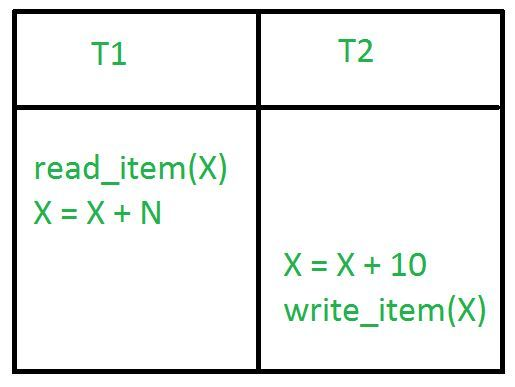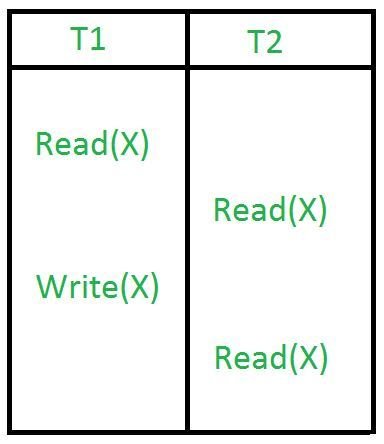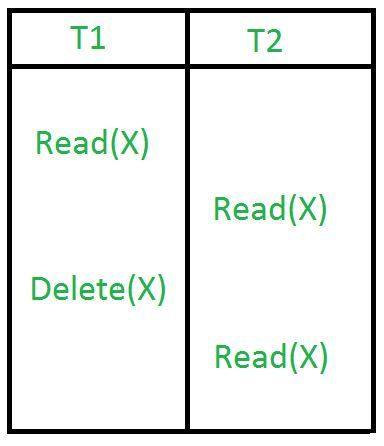If you want to make money online : Register now

# What are concurrent transactions ? Briefly discuss the problems encountered by concurrent trans

, ,

When multiple transaction execute concurrently in an uncontrolled or unrestricted manner, then it might lead to several problems. These problems are commonly referred to as concurrency problems in database environment. The five concurrency problems that can occur in database are:

```(i). Temporary Update Problem
(ii). Incorrect Summary Problem
(iii). Lost Update Problem

These are explained as following below.

1. Temporary Update Problem:
Temporary update or dirty read problem occurs when one transaction updates an item and fails. But the updated item is used by another transaction before the item is changed or reverted back to its last value.Example:
In the above example, if transaction 1 fails for some reason then X will revert back to its previous value. But transaction 2 has already read the incorrect value of X.
2. Incorrect Summary Problem:
Consider a situation, where one transaction is applying the aggregate function on some records while another transaction is updating these records. The aggregate function may calculate some values before the values have been updated and others after they are updated.Example:
In the above example, transaction 2 is calculating the sum of some records while transaction 1 is updating them. Therefore the aggregate function may calculate some values before they have been updated and others after they have been updated.
3. Lost Update Problem:
In the lost update problem, update done to a data item by a transaction is lost as it is overwritten by the update done by another transaction.Example:
In the above example, transaction 1 changes the value of X but it gets overwritten by the update done by transaction 2 on X. Therefore, the update done by transaction 1 is lost.
The unrepeatable problem occurs when two or more read operations of the same transaction read different values of the same variable.Example:
The phantom read problem occurs when a transaction reads a variable once but when it tries to read that same variable again, an error occurs saying that the variable does not exist.Example: